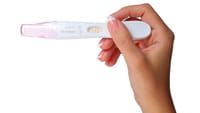0
Thanks

A few words of thanks would be greatly appreciated.

# How To Calculate Your Fertile Days (Ovulation)

Statistically, 20% of couples have difficulty conceiving a child; one primary element that couples seek to resolve is concentrating their efforts to conceive during the future mother's ovulation period.

This can be accomplished primarily by calculating one's ovulation period.## Calculating Fertility with a Normal Cycle

The best time to conceive is just after the ovulation phase, which spans the period of approximately four days before ovulation until approximately one day after it ends. The first day of the cycle is the first day of menstruation.

That is, four days before (i.e. the tenth day) and one day after (i.e. the 15th day) the 14th day of the cycle are ideal days for fertilization in a 28-day cycle.

If the cycle is greater than 35 days or less than 21 days, or if the menstrual cycle tends to be irregular, the results of this calculation may not be quite the same.

## Calculating Fertility with an Abnormal (Short or Long) Cycle

If the cycle is shorter — lasting only 21 days, for example — ovulation takes place on the seventh day from the first day of menstruation.

The calculation is performed by removing 14 days (i.e. the duration of the luteal phase, which does not vary) from the number of days that the cycle lasts (21 - 14 = 7).

If the cycle has a duration of 22 days: ovulation will take place on the 8th day (22 -14 = 8).

0
Thanks

A few words of thanks would be greatly appreciated.